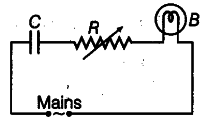# Capacitor C, a variable resistance R and a bulb B are connected in series

A capacitor C, a variable resistance R and a bulb B are connected in series to the AC mains in circuit as shown in the figure. The bulb glows with some brightness. How will the glow of the bulb change, if (i) a dielectric slab is introduced between the plates of the capacitor, keeping resistance R to be same; (ii) the resistance R is increased keeping the same capacitance?(i) As the dielectric slab is introduced between the plates of the capacitor, its capacitance will increase. Hence, the potential drop across the capacitor will decrease , i.e. V = Q/C
As a result, the potential drop across the bulb will increase as they are connected in series. Thus, its brightness will increase.
(ii) As the resistance R is increased, the potential drop across the resistor will increase. As a result, the potential drop across the bulb will decrease as they are connected in series. Thus, its brightness will decrease.